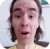FreeKB - How to use the foreach function with PHP and MySQL
How to use the foreach function with PHP and MySQLTo understand what the foreach function does, let's use an example where we have a MySQL table with a few columns of data.

• ID = 1
• Column1 = Hello world
• Column2 = How are you today

Before we introduce the foreach function, it is helpful to demonstrate how PHP will display data using a while loop with the mysqli_fetch_array function. Below is the code while the while loop. In this example, to display Hello world and How are you today, we must echo each row inside of the while loop. The Web browser will produce the data as one string of text:

Hello world How are you today

``````<?php

// Make a connection to MySQL

// Select all data from a table
\$select = "select * from tablename";

// Establish a query using the connection and select variables
\$select_query = mysqli_query(\$con, \$select);

// Use a while loop to create an array using the \$select_query variable
while (\$row = mysqli_fetch_array(\$select_query))

// echo each \$row
echo \$row['column1'];
echo \$row['column2'];

// End the while loop
}

?>``````

If we insert a foreach function inside of the while loop, we no longer need to each each row of data. This also will change the way the Web browser displays the data. Instead of displaying a single line of text for each array, every single \$row is separated by a page break. In this example, the Web browser would display the following:

Hello World
How are you today

``````<?php

// Make a connection to MySQL

// Select all data from a table
\$select = "select * from tablename";

// Establish a query using the connection and select variables
\$select_query = mysqli_query(\$con, \$select);

// Use a while loop to create an array using the \$select_query variable
while (\$row = mysqli_fetch_array(\$select_query))

// Create an array named \$data
\$data[] = \$row;

// Use the foreach function
foreach (\$data as \$row) {

// Echo each column
echo \$row['column1'];
echo \$row['column2'];

// Create a single page break after each record
echo "<br />";

// End the foreach loop
}

?>``````

We will never share your name or email with anyone. Enter your email if you would like to be notified when we respond to your comment.

Please enterin the box below so that we can be sure you are a human.